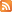Entries (RSS) -Comments (RSS)

### Chapter 2 Stash It Assignment

Chapter 2 Stash It Assignment 2011

In this chapter we worked with rational numbers. For your assignment you will have completed the following:

Blogged 2 questions.
Commented on at least 8 other questions. (4 marks)

Complete a Venn diagram showing our Real number system with definitions of each kind of number, its abbreviation, and examples. (4 marks)

Self assessment Stop Light Activity with examples to show that you know.
For Stop Light 2.2: “I can perform operations on rational numbers in decimal form.” You must show one of each of the following operations: +, -, ×, ÷
Each of the operations must demonstrate knowledge of using positives and negatives. (4 marks)

For Stop Light 2.2: “I can perform operations on rational numbers in fraction form.” You must show one of each of the following operations: +, -, ×, ÷
Each of the operations must demonstrate knowledge of using positives and negatives, and how to convert between mixed and improper fractions. (4 marks)

Problems of the Week question 3 and 4. (4 marks)